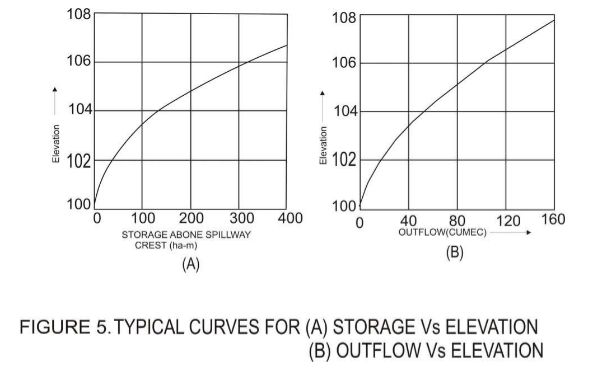### Rational Method of Derivation of Design Flood From Storm Studies and Application of Unit Hydrograph Principle

The steps involved, in brief, are:

1. Analysis of rainfall and run-off data for derivation of loss rates under critical conditions;

2. Derivation of unit hydrograph by analysis (or by synthesis, in cases where data are not available);

3. Derivation of the design storm; and

4. Derivation of design flood from the design storm by the application of the rainfall excess increments to the unit hydrograph.

The Maximum Water Level of a reservoir is obtained by routing the design flood through the reservoir and the spillway. This process of computing the reservoir storages,  storage volumes and outflow rates corresponding to a particular hydrograph of inflow is commonly referred to as flood routing.

The routing is carried out with the help of the following data,

1. Initial reservoir stage

2. The design flood hydrograph

3. Rate of outflow including the flow over the crest, through sluices or outlets and through power units

4. Incremental storage capacity of the reservoir

Typical values of the last two types of data, is shown in Figure 5.The routing of flood through the reservoir and the spillway is done by solving the continuity of flow within reservoir, which may simply be stated as:

Inflow to reservoir - Outflow to reservoir = Rise is water surface of the reservoir, that is an increase in the storage of the reservoir. That is,

Where, I is the inflow discharge (m3/s), O is the Outflow discharge (m3/s), s is the in storage volume (m3/s) in time interval t (h).

If the inflow hydrograph is known, then we may read out the inflow ordinates at every time interval. Suppose,

The following values are known:

I1 = Inflow (m3/s) at the beginning of a time interval

I2 = Inflow (m3/s) at the end of the time interval

O1= Outflow (m3/s) at the beginning of the time interval

S1= Total storage volume of the reservoir (m3/s) at the beginning of the time interval

And the unknown values are

S2= Total storage volume of the reservoir (m3/s) at the end of the interval O2= Outflow (m3/s) at the end of the time interval